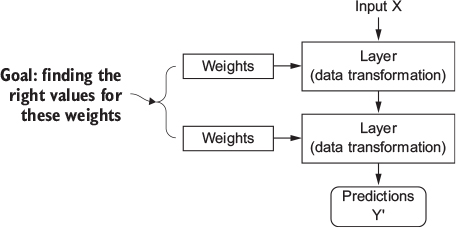## 15.7 Understanding how DL works (1)

• Reminder: ML about mapping inputs (e.g., images) to targets/outputs (e.g., label “cat”).. through observing many examples of inputs and targets/outputs
• Neural networks: do this input-to-target mapping via a deep sequence of simple data transformations (layers) [data transformations are learned by exposure to examples]
• But how does this happen concretely? See Figure 15.4.Figure 15.4: A neural network is parameterized by its weights (Chollet & Allaire, 2018, Fig. 1.7)

• Specification of what a layer does to its input data is stored in the layer’s weights (= a bunch of numbers) (see See Figure 15.4)
• Weights are also sometimes called the parameters of a layer
• Learning (in this context): Find set of values for the weights of all layers in a network, such that the network will correctly map example inputs to their associated targets
• Deep neural network can contain tens of millions of parameters
• Finding correct values for all of them may seem like a daunting task…